StatLect

# Transformation theorem

A transformation theorem is one of several related results about the moments and the probability distribution of a transformation of a random variable (or vector).## The transformation

Suppose that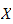is a random variable whose distribution is known.

Given a function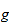, how do we derive the distribution of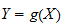?

## Formulae for one-to-one functions

If the functionis one-to-one (e.g., strictly increasing or strictly decreasing), there are formulae for the probability mass (or density) and the distribution function of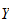.

These formulae, sometimes called transformation theorems, are explained and proved in the lecture on functions of random variables.

Their generalization to the multivariate case (whenis a random vector) are discussed in the lecture on functions of random vectors.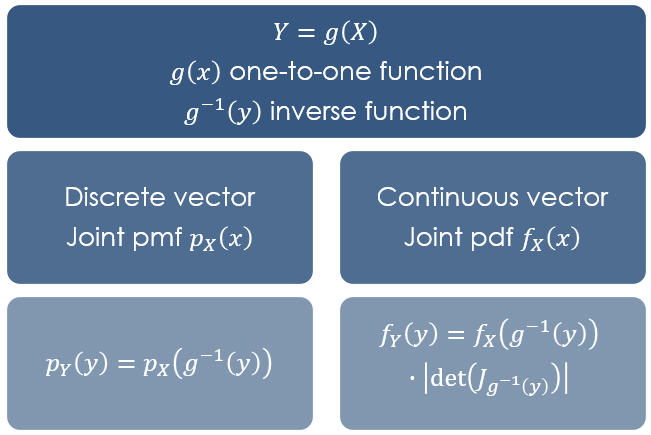## Law of the unconscious statistician

When the functionis not one-to-one and there are no simple ways to derive the distribution of, we can nonetheless easily compute the expected value and other moments of, thanks to the so-called Law Of the Unconscious Statistician (LOTUS).

The LOTUS, illustrated below, is also often called transformation theorem.

## LOTUS for discrete random variables

For discrete random variables, the theorem is as follows.

Proposition Letbe a discrete random variable and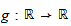a function. Define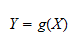Then,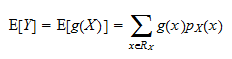where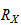is the support ofand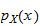is its probability mass function.

Note that the above formula does not require us to know the support and the probability mass function of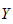, unlike the standard formula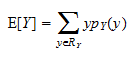## LOTUS for continuous random variables

For continuous random variables, the theorem is as follows.

Proposition Letbe a continuous random variable anda function. DefineThen,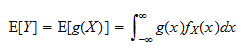where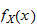is the probability density function of.

Again, the above formula does not require us to know the probability density function of, unlike the standard formula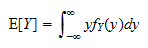## LOTUS for other moments

The LOTUS can be used to compute any moment of, provided that the moment exists: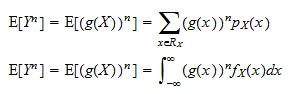## LOTUS for moment generating and characteristic functions

The LOTUS can be used to compute the moment generating function (mgf)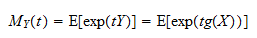The mgf completely characterizes the distribution of.

If we are able to calculate the above expected value and we recognize that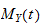is the joint mgf of a known distribution, then that distribution is the distribution of. In fact, two random variables have the same distribution if and only if they have the same mgf, provided the latter exists.

Similar comments apply to the characteristic function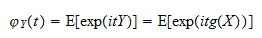## More details

More details about the transformation theorem can be found in the following lectures:

## References

Abadir, K.M. and Magnus, J.R., 2007. A statistical proof of the transformation theorem. The Refinement of Econometric Estimation and Test Procedures, Cambridge University Press.

Goldstein, J.A., 2004. An appreciation of my teacher, MM Rao. In Stochastic Processes and Functional Analysis (pp. 31-34). CRC Press.

Schervish, M.J., 2012. Theory of statistics. Springer Science & Business Media.

Previous entry: Test statistic

Next entry: Type I error